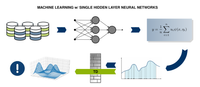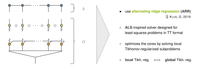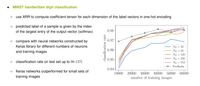# Fredholm Equations

The world of neural networks and machine learning has seen rapid advancements in recent years. Neural networks have become a go-to tool for various applications, from image recognition to natural language processing. However, the process of training neural networks can be challenging, involving complex optimization techniques. In this blog post, we'll explore a novel and mathematically transparent approach to function approximation and the training of large, high-dimensional neural networks using Fredholm integral equations . This approach is based on the approximate least-squares solution of associated Fredholm integral equations of the first kind, Ritz-Galerkin discretization, Tikhonov regularization, and tensor-train methods.Efficient and reliable methods for training neural networks are essential for their success. Traditional techniques like stochastic gradient methods have been around for decades and are widely used. However, they can be complex and require meticulous parameter tuning for convergence. This blog post introduces a fresh perspective on training neural networks using integral equations.

The core idea of this approach is to treat neural networks as Monte Carlo approximations of integrals over a parameter domain $Q$, assuming that the parameters are uniformly distributed. This leads to the concept of "Fredholm networks," which provide a unique way to approximate functions. Training these networks involves solving linear Fredholm integral equations of the first kind for a parameter function:

 Fredholm training problem: Find $u \in L^2(Q)$ such that $\mathcal{J}(u) \leq \mathcal{J}(v)$ for all $v \in L^2(Q)$with $\mathcal{J}(v) = \lVert F - \mathcal{G} v \rVert_\pi^2 = \int_X (F(x) - \mathcal{G} v (x))^2 d \pi(x)$ and $\mathcal{G} v = \int_Q \psi(\,\cdot\,,\eta) v(\eta) d \eta$.

To address the ill-posedness of the Fredholm training problem, we employ Ritz-Galerkin discretization and Tikhonov regularization. These techniques help approximate the solution to the integral equations, making the approach more practical for real-world applications:

 regularized Fredholm training problem: Find $u \in S$ such that $\mathcal{J}_\varepsilon(u) \leq \mathcal{J}_\varepsilon(v)$ for all $v \in S$with $\mathcal{J}_\varepsilon (v) = \mathcal{J} (v) + \varepsilon \lVert v \rVert^2$and $S := \mathrm{span}\,\{\varphi_1, \dots, \varphi_p\} \subset L^2 (Q)$.

One of the highlights of this approach is the use of tensor-train methods for solving large linear systems. By considering the algebraic formulation of the regularized Fredholm training problem and employing alternating linear schemes, we can efficiently handle high-dimensional parameter domains.To demonstrate the effectiveness of the Fredholm approach, we applied it to three well-established test problems: regression and classification tasks. The experiments included the UCI banknote authentication dataset, concrete compressive strength prediction, and the MNIST dataset. The results showed that Fredholm-trained neural networks are highly competitive with state-of-the-art methods, even without problem-specific tuning.In conclusion, the Fredholm integral equation approach offers a promising alternative for training neural networks and function approximation. Its transparency and competitive performance make it a valuable addition to the toolbox of machine learning practitioners. As the field continues to evolve, approaches like this open new avenues for efficient and effective training of neural networks. For more details, we refer to our preprint:

 P. Gelß, A. Issagali, R. Kornhuber. Fredholm integral equations for function approximation and the training of neural networks. arXiv: 2303.05262